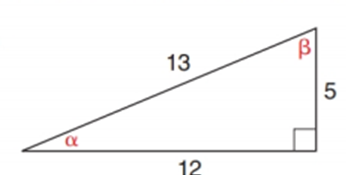Chapter 11.2, Problem 8EElementary Geometry For College St...

7th Edition
Alexander + 2 others
ISBN: 9781337614085

Solutions

Chapter
SectionElementary Geometry For College St...

7th Edition
Alexander + 2 others
ISBN: 9781337614085
Textbook Problem

Using the right triangle from Exercise 1, show that sin 2 α + cos 2 α = 1 Exercises 1.To determine

To find:

To show that sin2α+cos2α=1.

Explanation

Consider the following figure,

General formula for cosine and sine ratio is given below,

From the given figure 13 is the hypotenuse value since it is opposite to right angle.

Then 12 is the length of the leg adjacent to α and 5 is the length of leg opposite to α.

Therefore,

cosα=1213 and sinα=513

Consider sin2α+cos2α=1.

Substitute the values of cosα=1213 and sinα=513 in the above equation to get the following,

Still sussing out bartleby?

Check out a sample textbook solution.

See a sample solution

The Solution to Your Study Problems

Bartleby provides explanations to thousands of textbook problems written by our experts, many with advanced degrees!

Get Started

Find the average value of f on [0, 8].

Single Variable Calculus: Early Transcendentals, Volume I

11. Use a normal approximation to a binomial distribution to find the probability that .

Mathematical Applications for the Management, Life, and Social Sciences

Evaluate . e + 1

Study Guide for Stewart's Single Variable Calculus: Early Transcendentals, 8th